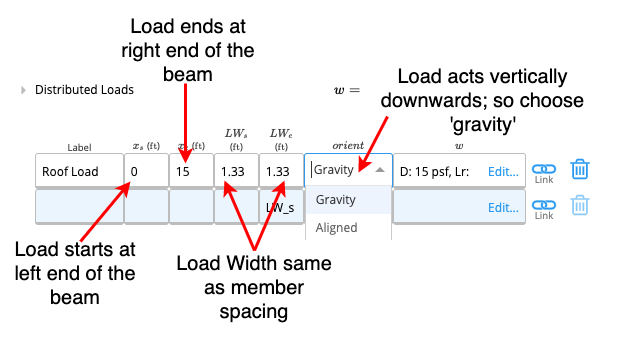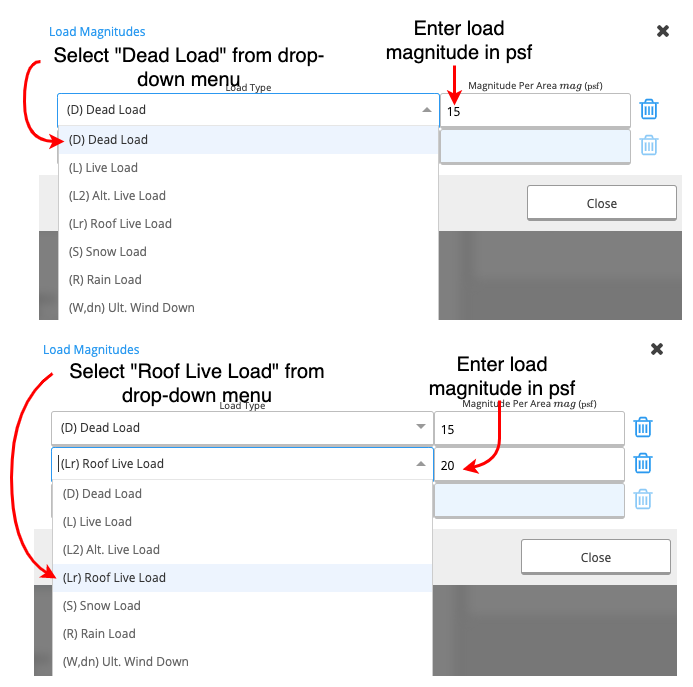How to Enter Distributed Loads (Imperial Units)

In ClearCalcs there is a designated table for Distributed Loads under the Loads section. This table is accompanied by a diagram of the structural component that is being analysed, which updates in real-time and indicates the loads you have entered.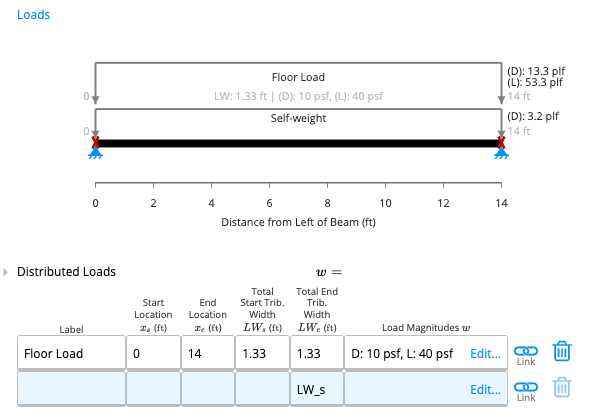This article will discuss the input parameters in the Distributed Loads table and gives some worked examples on how to input distributed loads into ClearCalcs for structural design applications.

Input Parameters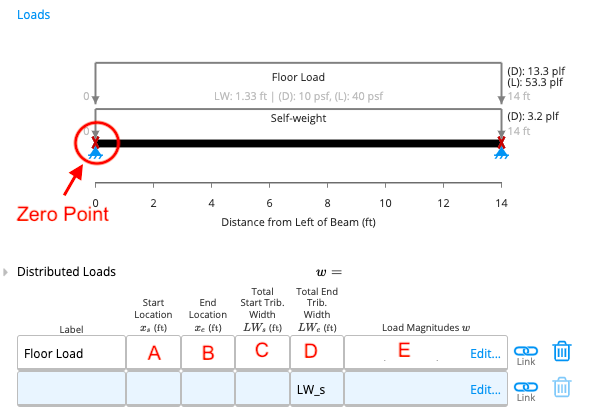A. Start Location

Start Location refers to the starting point of the load on the beam as measured from the left end of the beam (from the zero point). This parameter needs to be in feet, alternatively, conversion formulae can also be entered into the cell for convenience (similar to Excel).

B. End Location

End Location refers to the point on the beam at which the distributed load ceases to act. This parameter is also measured from the left end (zero point) of the beam and needs to be in feet. If the end location is also the right end of the beam, you can input 'L' for convenience (see Example 1).

C-D. Tributary Width / Load Width

Every structural element has an area from which it obtains its load. As with any other area, this can also be expressed as a product of length and width. The length of this area is usually either the length of the element itself or the length of the distributed load (B-A from the table). Tributary width is simply the width of this area.

How to find Tributary Width?

To better illustrate this concept, consider a floor that is supported by members spaced 's' ft apart.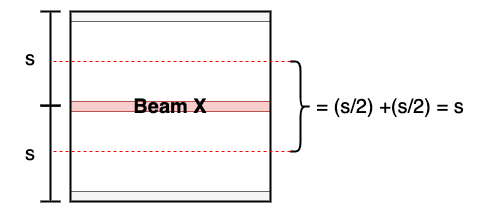Consider beam X, where its tributary area has the same length as the beam and the tributary width is 's'. So for most cases, the tributary width is the same as member spacing. Notice that the tributary area is not always a rectangle and can instead take on a triangular shape. In such instances, the starting and ending tributary widths will be different.

C. Total Start Tributary Width

Measured in feet, the total start tributary width refers to the width of the tributary area at the starting point of the distributed load.

D. Total End Tributary Width

Measured in feet, the total end tributary width refers to the width of the tributary area at the ending point of the distributed load.

When you click on this cell, a new table will appear (see below).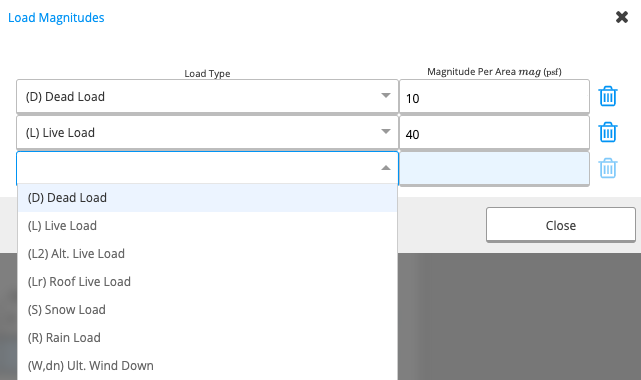• Second Column: You can input the magnitude of the load in Pounds per Square Foot (PSF).

Example 1

Input a live load of 40psf on a floor joist with a member spacing of 1.33ft.

Method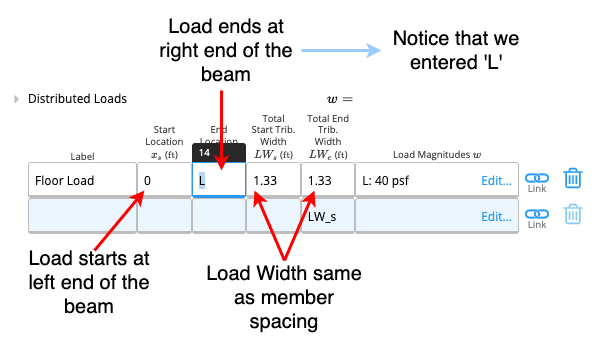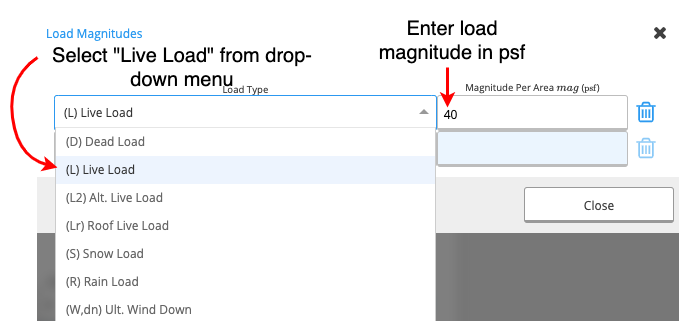Example 2

Input a dead load of 10psf on a ceiling joist with a member spacing of 2ft.

Method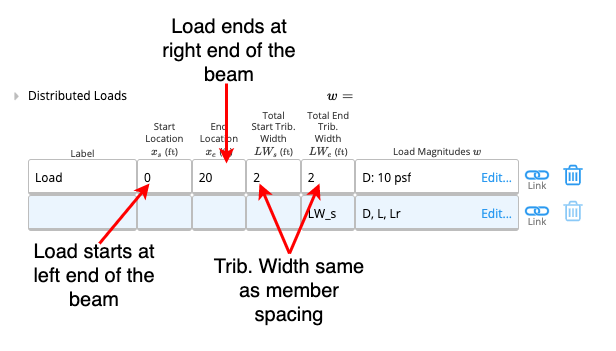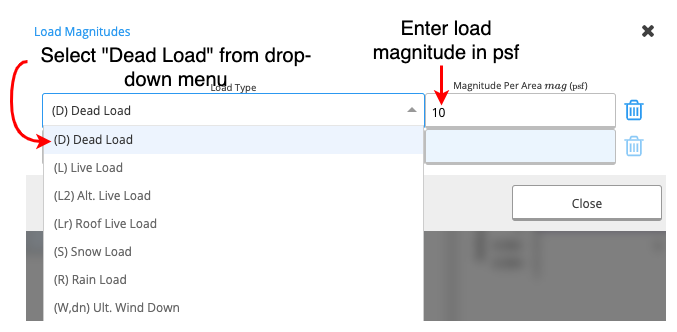Example 3

Input a roof live load of 20psf and a dead load of 15psf on a rafter with 1.33ft member spacing.

Note:

In the calculator for rafters, you will notice that there's an additional column in the distributed loads table (as shown in the image below).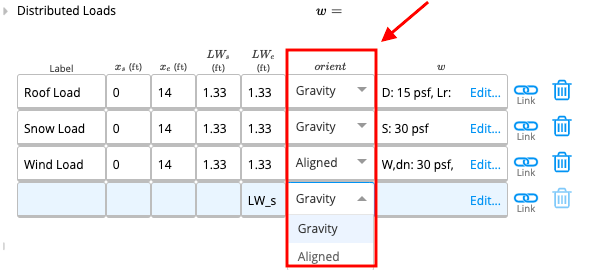This column refers to the orientation of the line of action of the specified load. There is a drop-down menu from which you can choose either gravity or allied.

Gravity: The load always acts vertically downwards. e.g. weight force

Allied: The load acts perpendicular to the surface of the structural element. e.g. wind

Method